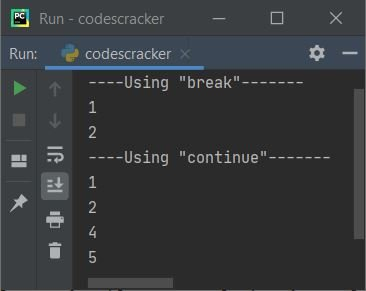# Difference between break and continue in Python

This tutorial is created to clear your doubts about one of the famous comparisons between two conditional statements in the Python programming language, namely the break and continue statements.

## break vs. continue in Python

The table given below differentiates break and continue keywords:

break continue
leaves the loop jumps for the next iteration
skips the remaining execution of the loop. skips execution of the remaining statement(s) inside the loop for the current iteration.
If the condition always evaluates to true, it is useful. useful if one wants to skip execution of some statement(s) inside the loop for any particular iteration.

## break vs. continue example in Python

I'm sure that after understanding the example program given below, you will have a complete understanding of the break vs. continue keywords in Python. In Python programming, this is how these two keywords or statements are used:

```nums = [1, 2, 3, 4, 5]

print("----Using \"break\"-------")
for n in nums:
if n == 3:
break
print(n)

print("----Using \"continue\"-------")
for n in nums:
if n == 3:
continue
print(n)```

This program produces the output as shown in the snapshot given below:As you can see from the above program, the first block of code is:

```for n in nums:
if n == 3:
break
print(n)```

prints "1, 2." Because when the value of n becomes 3, the condition "n == 3" or "3 == 3" evaluates to be true, and using break, the execution of the loop gets terminated. Whereas the second part is the following block of code:

```for n in nums:
if n == 3:
continue
print(n)```

prints all the numbers except 3. Because when the condition "n == 3" evaluates to be true, therefore using "continue," the program flow jumps to the next iteration of the loop, and the remaining statement(s) after the keyword "continue," that is, print(n), get skipped for that iteration.

Python Online Test

« Previous Tutorial Next Tutorial »